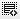## A question from the quiz.0
If you took \$20 million as debt to fund a \$200 million project. Assuming 10% interest on the debt and returns of \$500 million in 5 years. What is your ROI?

My solution:

Debt= \$20 million
Own investment = \$180 million

Interest @ 10% for 5 years = \$10 million.
Return = 500-20(debt)-10(interest)= \$470 million.

ROI= 470/180. This is not equal to 61 %. Please tell me what am I doing wrong.0

In this question Debt is 20 million and interest 10% so after 5 year you have to return 30 million....if expected return after 5 years is 500 million then left over is 470 million and your investment is 180 million so ROI = 470/180=261%

Click on thisicon to add code snippet.